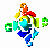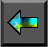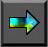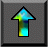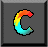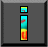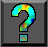Next: 4.7 Summary Up: 4 Putting Components Together Previous: 4.5 Case Study: Tuple Space

# 4.6 Case Study: Matrix Multiplication

In our third case study,   we use the example of matrix-matrix   multiplication to illustrate issues that arise when developing data   distribution neutral libraries. In particular, we consider the   problem of developing a library to compute C = A.B , where A , B , and C are dense matrices of size N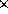N . (A dense matrix is a matrix in which most of the entries are nonzero.) This matrix-matrix multiplication involves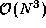operations, since for each elementof C , we must compute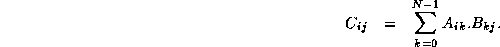We wish a library that will allow each of the arrays A , B , and C to be distributed over P tasks in one of three ways: blocked by row, blocked by column, or blocked by row and column. This library may be defined with a subroutine interface suitable for sequential composition in an SPMD program or with a channel interface suitable for parallel or concurrent composition. The basic algorithmic issue remains the same: Does the library need to incorporate different algorithms for different distributions of A , B , and C , or should incoming data structures be converted to a standard distribution before calling a single algorithm?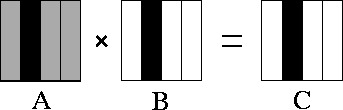Figure 4.10: Matrix-matrix multiplication A.B=C with matrices A , B , and C decomposed in one dimension. The components of A , B , and C allocated to a single task are shaded black. During execution, this task requires all of matrix A (shown stippled).

## 4.6.1 Parallel Matrix-Matrix Multiplication

We start by examining algorithms for various distributions of A ,   B , and C . We first consider a one-dimensional, columnwise decomposition in which each task encapsulates corresponding columns from A , B , and C . One parallel algorithm makes each task responsible for all computation associated with its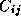. As shown in Figure 4.10, each task requires all of matrix A in order to compute its.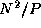data are required from each of P-1 other tasks, giving the following per-processor communication cost:Note that as each task performs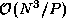computation, if N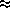P , then the algorithm will have to transfer roughly one word of data for each multiplication and addition performed. Hence, the algorithm can be expected to be efficient only when N is much larger than P or the cost of computation is much larger than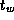.Figure 4.11: Matrix-matrix multiplication A.B=C with matrices A ,   B , and C decomposed in two dimensions. The components of A , B , and C allocated to a single task are shaded black. During execution, this task requires corresponding rows and columns of matrix A and B , respectively (shown stippled).

Next, we consider a two-dimensional decomposition of A , B , and C . As in the one-dimensional algorithm, we assume that a task encapsulates corresponding elements of A , B , and C and that each task is responsible for all computation associated with its. The computation of a single elementrequires an entire row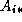and column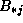of A and B , respectively. Hence, as shown in Figure 4.11, the computation performed within a single task requires the A and B submatrices allocated to tasks in the same row and column, respectively. This is a total of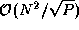data, considerably less than in the one-dimensional algorithm.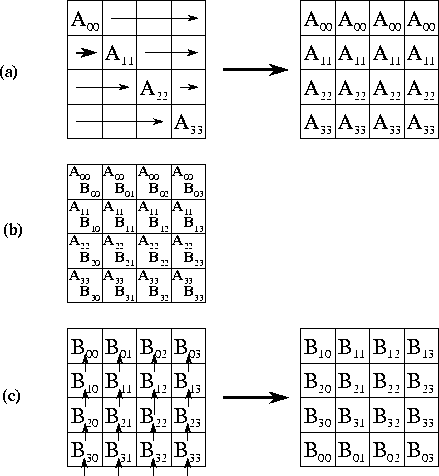Figure 4.12: Matrix-matrix multiplication algorithm based on two-dimensional decompositions. Each step involves three stages: (a) an A submatrix is broadcast to other tasks in the same row; (b) local computation is performed; and (c) the B submatrix is rotated upwards within each column.

To complete the second parallel algorithm, we need to design a strategy   for communicating the submatrices between tasks. One approach is for each task to execute the following logic (Figure 4.12):

```
set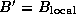for  j
=0 to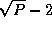in each row  i
, the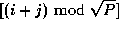th task broadcasts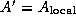to the other tasks in the row

accumulate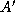.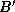sendto upward neighbor

endfor

```

Each of the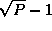steps in this algorithm involves a broadcast totasks (for A' ) and a nearest-neighbor communication (for B' ). Both communications involvedata. Because the broadcast can be accomplished in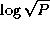steps using a tree structure, the per-processor communication cost is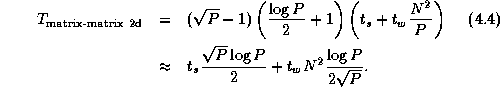Notice that because every task in each row must serve as the root of a broadcast tree, the total communication structure required for this algorithm combines a hypercube (butterfly) structure within each row of the two-dimensional task mesh and a ring within each column.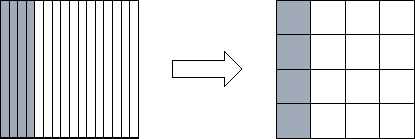Figure 4.13: Reorganizing from a one-dimensional to a one-dimensional decomposition of a square matrix when P=16. Shading indicates one set of four tasks that must exchange data during the reorganization.

## 4.6.2 Redistribution Costs

Comparing Equations 4.3 with 4.4,   we see that the two-dimensional decomposition yields the more efficient parallel algorithm. Does this mean that our parallel library should convert input arrays decomposed in one dimension to a two-dimensional decomposition before performing the matrix multiplication? To answer this question, we need to know the cost of the reorganization. The communication costs associated with the reorganization of a single array are as follows; each task exchanges data withother tasks, with each message having size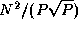(Figure 4.13):If A , B , and C are all decomposed in one dimension, we must perform three such conversions. This gives a worst-case total communication cost for reorganization and multiplication using the two-dimensional algorithm of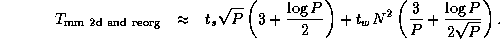Comparing this expression with Equation 4.3, we see that the algorithm that reorganizes data structures to a 2-D decomposition before performing the multiplication will be more efficient than an algorithm that does not, when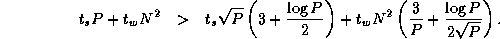This condition holds for all except small P . Hence, we conclude that our parallel matrix multiply library should convert to a two-dimensional decomposition before performing computation, as follows.

```
procedure matrix_multiply(A, B, C)

begin

if 1d_distributed(A) then reorg_to_2d(A)

if 1d_distributed(B) then reorg_to_2d(B)

2d_matrix_multiply(A, B, C)

if 1d_distributed(C) then reorg_to_1d(C)

end

```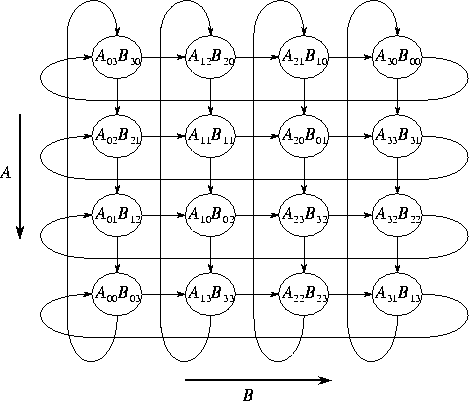Figure 4.14: Layout of the A and B matrices in the systolic matrix-matrix multiplication algorithm for a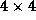task mesh. The arrows show the direction of data movement during execution of the systolic algorithm.

## 4.6.3 A Systolic Algorithm

We still have not said the last word about the ideal data distribution for matrix-matrix multiplication! An alternative algorithm   allows the broadcast operations used in the preceding algorithm to be   replaced with regular, nearest-neighbor (``systolic'') communications. However, data must be distributed among tasks in a different fashion. As before, we assume that A , B , and C are decomposed into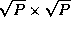submatrices. Each task (i,j) contains submatrices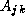,, and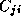, where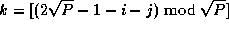. This data layout is illustrated in Figure 4.14.

Computation proceeds in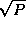steps. In each step, contributions to C are accumulated in each task, after which values of A move down and values of B move right. The entire computation requires a total of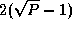messages per task, each of size, for a cost of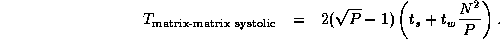Communication costs are less by a factor of aboutthan in Equation 4.4. Again, this benefit must be weighed against the cost of converting matrices A , B , and C into the layout required by this algorithm. This analysis is left as   an exercise.Next: 4.7 Summary Up: 4 Putting Components Together Previous: 4.5 Case Study: Tuple Space